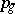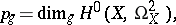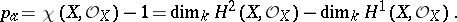# Genus of a surface

(diff) ← Older revision | Latest revision (diff) | Newer revision → (diff)

A numerical birational invariant of a two-dimensional algebraic variety defined over an algebraically closed field. There are two different genera — the arithmetic genus and the geometric genus. The geometric genusof a complete smooth algebraic surfaceis equal toi.e. to the dimension of the space of regular differential-forms (cf. Differential form) on. The arithmetic genusof a complete smooth algebraic surfaceis equal toThe geometric and arithmetic genera of a complete smooth algebraic surfaceare related by the formula, whereis the irregularity of, which is equal to the dimension of the space of regular differential-forms on.

How to Cite This Entry:
Genus of a surface. Encyclopedia of Mathematics. URL: http://encyclopediaofmath.org/index.php?title=Genus_of_a_surface&oldid=16127
This article was adapted from an original article by Vik.S. Kulikov (originator), which appeared in Encyclopedia of Mathematics - ISBN 1402006098. See original article